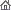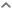Сhair of Accounting & Audit.UIBl
 Вы вошли как Гость | Группа "Гости"Приветствую Вас Гость | RSS
 Меню сайтаКатегории разделаМои статьи  Статьи преподавателей Публикации преподавателей УМБ Статьи студентов Статьи студентов Статьи магистрантов Статьи магистрантов Статьи докторантов Статьи докторантов III Научная конференция "Молодежь в науке" при Форуме Первого ПрезидентаНаш опросОцените мой сайт Отлично Хорошо Неплохо Плохо Ужасно Всего ответов: 58СтатистикаОнлайн всего: 1 Гостей: 1 Пользователей: 0Football matches forecasting using three mathematical statistical methods
 Football can not be ignored by scientific research, including mathematical sciences. The crucial issue here is an adequate assessment of the sport event and the forecast of performance of teams.       We will focus on the basic indicators characterizing the entertainment, the dynamism, the level of competition and the balance of team forces - on the scoring performance.      We would try to compare several mathematical methods associated with the statistics that can serve as methods to determine the victory of any team in the match with an opponent, we compare these methods and in our opinion, we will choose the best of the proposals. In order to compare the effectiveness of teams or players who had a different number of matches, average performance is the most appropriate tool of comparison.       However, there are some pitfalls that can lead to the fact that this tool is, figuratively speaking, "will be spoiled", not leading to an objective comparison, but distorting the real picture.       However, in the characteristics of the various football tournaments, teams or individual players the concept of "average performance" is used quite often.       It would be better to speak of "the arithmetic mean of the impact", i.e. the number of goals scored divided by the number of matches. The meaning of "average performance" - is the most likely outcome of an arbitrarily chosen match. Average performance provides us with a more or less accurate guide to our expectations of the number of goals scored in every match.      However, using the arithmetic mean is often not sufficiently informative. Let's consider an example. Table 1 Results of the Premier League (England) 2010-11, team – Chelsea (1-3 tours) Tour number 1 2 3 Number of goals 6 6 2 Average effectiveness of team for the 1-3 tours is calculated as (6+6+2)/3 = 5 goals per match Table 2 Results of the Premier League (England) 2010-11, team – Chelsea (5-7 tours) Tour number 5 6 7 Number of goals 4 0 2 Average effectiveness of team for the 1-3 tours is calculated as (4+0+2)/3 = 2 goals per match.   If you hear about the number of average effectiveness and you don’t know anything more, you will go to the stadium with foretaste to see unbelievable goals. But in reality this simple method doesn’t work. In 1-3 tours team Chelsea has more effective usage of its tactics and power, but during 5-7 tours we see such appropriateness which misleads us. Considering this method, there is not enough information about teams and footballers, because we need to make a clear picture of last matches to make some clear forecast. In our opinion, this method is not suitable in any case for football forecasting. What about correlation and regression method of analysis? Let’s use an example.Using this paired correlation (between wins and goals parameters) we have calculated the relation equation by the method of least squares, by decision of the equation system. With the help of received data we have calculated the correlation coefficient for characteristic of strength degree of connection in case of linear dependence; by this parameter we can see that the qualitative estimation of connection degree from the Chedok scale is characterized as moderate connection.     The coefficient of determination shows the dependence degree equally to 0.0044 % of the resulting parameter from the change of factorial parameter. Also we tried to do another calculation.From this calculation we can see that the correlation coefficient for characteristic of strength degree of connection in case of linear dependence equals to zero that is show us that there is no dependence between ‘goals and loses’ or there is a little dependence. From these two examples, it is necessary to say that using correlation and regression method of analysis for football matches’ forecasts cannot give us a clear picture of dependences and also the picture of team who will win one or another match, of course, there is a little level of probability, as we think.              We want to talk about another method, the forecast probability of which equals to 65%-70%. Using the Bayes formula, we can calculate the result of the match. This formula looks like:For the first view, there is incomprehensibility. But using "live” example, we think it will be clear. So, we took into account three examples. First of all, bringing in the series of conceptions and designations: • A – the match took place (event);     • H1 – hypothesis that home (1st team) will win;     • H2 – hypothesis that guest (2nd team) will win;     • H3 – hypothesis that between these two teams will be draw game;      • PH1(A) – statistical probability, that is ratio between quantity of victory matches at home to the common quantity of home matches;    • PH2 (A) - statistical probability, that is ratio between quantity of victory guest matches to the common quantity of guest matches;     • PH3 (A) – summary statistical probability that is (the quantity of draw games of the 1st team + the quantity of draw games of the 2nd team) / common quantity of the matches of one of teams.     Our goal is to determine or appreciate (to forecast), for example, the win of the 1st team or to find Pa (H1). For the achieving, we use statistical data (in our examples – for current season of the Premier League – England, 2010-2011).     In each example P(H1) = P(H2) = P (H3) = 1/3 (similar probability of events – win 1, win 2, draw game) First example is about weather bounded match in 16 tour of the Premier League (England): Blackpool – Manchester United Let’s suppose this match’s outcome using calculation of the Bayes formula.     PH1 (A) = 2/6=0.33 PH2 (A) = 2/9=0.22 PH3 (A) = 4 (Blackpool’s draw games) + 7 (Manchester United’s draw games) / 15 (common quantity of tours) = 0.73 P (A) = 0.33*0.33+0.33*0.22+0.33*0.73 = 0.4224 Pa (H1) = 0.33*0.33/0.4224 = 0.25781 (approx. 26%)    Pa (H2) = 0.33*0.22/0.4224 = 0.17181 (approx. 17%)    Pa (H3) = 0.33*0.73/0.4224 = 0.57031 (approx. 57%)    That is 26 % - Blackpool will win Manchester United                  17 % - Manchester United will win Blackpool                  57 % - will be draw game     Second example is about match that will be in 17 tour of the Premier League (England): Manchester United – Arsenal PH1 (A) = 6/8=0.75 PH2 (A) = 1/7=0.14286 PH3 (A) = 7 (Manchester United’s draw games) + 7 (Arsenal’s draw games) / 15 (common quantity of tours) = 0.6 P (A) = 0.3*0.75+0.3*0.14286+0.3*0.6 = 0.447858 Pa (H1) = 0.3*0.75/0.447858 = 0.50239 (approx. 50%) Pa (H2) = 0.3*0.14286/0.447858 = 0.0957 (approx. 10%) Pa (H3) = 0.3*0.6/0.447858 = 0.401913 (approx. 40%)    That is 50 % - Manchester United will win Arsenal                 10 % - Arsenal will win Manchester United                 40 % - will be draw game    Third example is about match that will be in 17 tour of the Premier League (England):     Tottenham – Chelsea PH1 (A) = 4/8=0.5 PH2 (A) = 3/8=0.375 PH3 (A) = 5 (Tottenham’s draw games) + 3 (Chelsea’s draw games) / 16 (common quantity of tours) = 0.5 P (A) = 0.3*0.5+0.3*0.375+0.3*0.5 = 0.4125 Pa (H1) = 0.3*0.5/0.4125 = 0.3636 (approx. 36%)    Pa (H2) = 0.3*0.375/0.4125 = 0.2727 (approx. 27%)    Pa (H3) = 0.3*0.5/0.4125 = 0.3636 (approx. 36%)     That is 36 % - Tottenham will win Chelsea                  28 % - Chelsea will win Tottenham                  36 % - will be draw game     In considering of the Bayes formula’s method, we see the regularity that we use previous home and guest matches data of the team where coming match will be as well as draw games of each team of coming match meeting.     One of the most important thing in this method, is that home match team has higher probability to win match than guest team. One might, we give proper weigh to previous wins during guest and home matches. In our opinion, this method is more effective than other ones. Because the forecast probability of Bayes formula equals to 65%-70%, that is odds appreciate as 7/10. Total-lot forecast does not exist, there is no method which can give such probability. Discussion in the Forum is here: Football Forecasting Statistics Источник: http://www.uib.ucoz.com Категория: Статьи студентов | Добавил: LilyK (09.12.2010) | Автор: LilyПросмотров: 795 | Комментарии: 7 | Рейтинг: 0.0/0 | - Оценить - Отлично Хорошо Неплохо Плохо Ужасно
Всего комментариев: 70Спам 7 Alla   (26.12.2010 20:34) The methods are really working, but... The author didn't mentioned different internal(such as psychological) and external (the factor of climatic change- players from Argentina having match in China) factors. The probability of occurrence decrease. Nevertheless, the second method is impressive.0Спам 6 Назикжан Монтаева   (18.12.2010 18:34) it's amazing scientific reasearch!!! you shouldn't stop doing it =) May be some day we will be able to predict even not only quantity of goals but even who will make this goaals =)0Спам 5 Anastasiya Rogozhina   (17.12.2010 21:46) This article is a great example, how students can use their statistical knowledge. It was interesting to read about both methods of forcasting. But, as for me, the second method is more impressive, because of the accurate results.0Спам 4 Anastasiya Deshevikh   (17.12.2010 16:11) Oh yes, the second method is very interesting! I'm sure these methods of calculating, always will be interesting for football fans. After I learned of the Bayes formula, I want to test it in practice. No need to be psychic, you just need to know the statistics and anticipate events on the basis of calculations.0Спам 3 Anara Kipatova   (16.12.2010 14:47) Very interesting article, but it's not always the truth, that home match team has higher probability to win match than guest team, it depends on the command more. For those who for a long time in football, probability of a victory is higher, than for a young command. Although statistical method is interesting thing for estimation)0Спам 2 Yekaterina Sukhova   (14.12.2010 21:55) Wow, that was a good piece of reading, thanks to the author. Really thought-provoking. Football, unfortunately, depends not only on historical data, but pretty much on team members, who, as we know, can be traded, and this factor (oh no, I don't know how) should be taken into consideration. If you find a way to do so (maybe somehow considering personal "productivity" of each player separately, which is a HUGE work, really time- and effort-consuming), it would be a great step forward. All aforesaid is just a humble opinion, of course) Thanks again, Yekaterina Sukhova0Спам 1 Kanat   (14.12.2010 09:00)Now we have the results of the predicted games. Manchester United (50%) won Arsenal 1:0 Tottenham played draw game with Chelsea. 1:1 In the last situation it was not so clear as we had equal probabilities (36%) for two situations: draw game or Tott's victory. Next step is to define which of the equals more probable.

Имя *:
Email *:
Код *:
 Форма входаПоискCopyright MyCorp © 2019 Сделать бесплатный сайт с uCoz